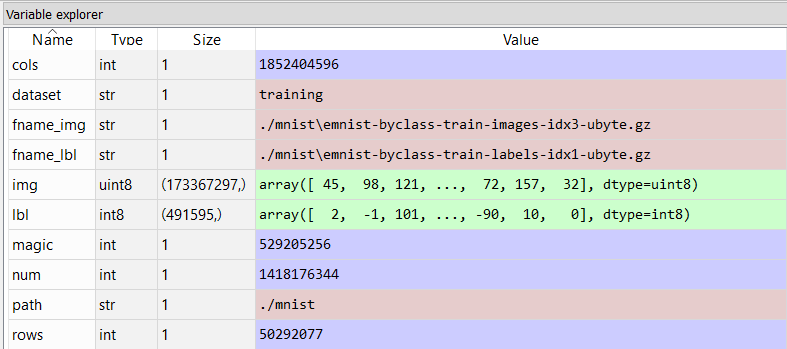{{ message }}

Instantly share code, notes, and snippets.

# akesling/mnist.py

Last active Jan 25, 2021
 import os import struct import numpy as np """ Loosely inspired by http://abel.ee.ucla.edu/cvxopt/_downloads/mnist.py which is GPL licensed. """ def read(dataset = "training", path = "."): """ Python function for importing the MNIST data set. It returns an iterator of 2-tuples with the first element being the label and the second element being a numpy.uint8 2D array of pixel data for the given image. """ if dataset is "training": fname_img = os.path.join(path, 'train-images-idx3-ubyte') fname_lbl = os.path.join(path, 'train-labels-idx1-ubyte') elif dataset is "testing": fname_img = os.path.join(path, 't10k-images-idx3-ubyte') fname_lbl = os.path.join(path, 't10k-labels-idx1-ubyte') else: raise ValueError, "dataset must be 'testing' or 'training'" # Load everything in some numpy arrays with open(fname_lbl, 'rb') as flbl: magic, num = struct.unpack(">II", flbl.read(8)) lbl = np.fromfile(flbl, dtype=np.int8) with open(fname_img, 'rb') as fimg: magic, num, rows, cols = struct.unpack(">IIII", fimg.read(16)) img = np.fromfile(fimg, dtype=np.uint8).reshape(len(lbl), rows, cols) get_img = lambda idx: (lbl[idx], img[idx]) # Create an iterator which returns each image in turn for i in xrange(len(lbl)): yield get_img(i) def show(image): """ Render a given numpy.uint8 2D array of pixel data. """ from matplotlib import pyplot import matplotlib as mpl fig = pyplot.figure() ax = fig.add_subplot(1,1,1) imgplot = ax.imshow(image, cmap=mpl.cm.Greys) imgplot.set_interpolation('nearest') ax.xaxis.set_ticks_position('top') ax.yaxis.set_ticks_position('left') pyplot.show()

###j5scott commented Oct 23, 2016

 Greys is an error, line 49 :( how to fix?

###RobertHerreraEECS commented Oct 24, 2016

 @j5scott try plt.cm.gray

###SaraUmut commented Mar 17, 2017

 sorry for asking but I am stuck on: how can I view an image using this code?

###marianokamp commented Mar 28, 2017

 @BigHopes, after putting the unzipped files into ./mnist below my notebook this worked for me in Jupyter:Also, to get it to work with Python 3, three changes were necessary. Add braces to line 24, xrange to range, and maybe one more thing that I now can't remember.

###nwjlyons commented Mar 29, 2017

 In order to get the show function to work you need to pass the second element of the tuple. eg. ``````mnist = read("training") image = mnist.next() show(image) `````` If you are curious (like me) about how the numbers in the matrix make up the image, the function below will show that. ``````def ascii_show(image): for y in image: row = "" for x in y: row += '{0: <4}'.format(x) print row ``````###cyanzhqq commented Apr 14, 2017 • edited

 This script is very helpful. For python3, I think we should use image = mnist.next()

###krapes commented Jun 21, 2017

 Does anyone know if these functions are compatible with the EMNIST dataset? https://www.nist.gov/itl/iad/image-group/emnist-dataset I would like to use it to parse the files but the read function keeps giving me this error: ` img = img.reshape(len(lbl), rows, cols) ValueError: total size of new array must be unchanged` Full function: ``````def read(dataset = "training", path = "."): if dataset is "training": fname_img = os.path.join(path, 'emnist-byclass-train-images-idx3-ubyte.gz') fname_lbl = os.path.join(path, 'emnist-byclass-train-labels-idx1-ubyte.gz') elif dataset is "testing": pass fname_img = os.path.join(path, 'emnist-byclass-test-images-idx3-ubyte.gz') fname_lbl = os.path.join(path, 'emnist-byclass-test-labels-idx1-ubyte/gz') else: raise ValueError( "dataset must be 'testing' or 'training'") with open(fname_lbl, 'rb') as flbl: magic, num = struct.unpack(">II", flbl.read(8)) lbl = np.fromfile(flbl, dtype=np.int8) print(len(lbl)) with open(fname_img, 'rb') as fimg: magic, num, rows, cols = struct.unpack(">IIII", fimg.read(16)) img = np.fromfile(fimg, dtype=np.uint8) img = img.reshape(len(lbl), rows, cols) get_img = lambda idx: (lbl[idx], img[idx]) for i in range(len(lbl)): yield get_img(i) ``````###wendao commented Jul 26, 2017

 @krapes gunzip those gz files, it works fine with EMNIST

###ghost commented Aug 5, 2017

 getting error while executing the above code: IOErrorTraceback (most recent call last) in () ----> 1 train_data = list(read(dataset='training', path='.')) in read(dataset, path) 25 26 # Load everything in some numpy arrays ---> 27 with open(fname_lbl, 'rb') as flbl: 28 magic, num = struct.unpack(">II", flbl.read(8)) 29 lbl = np.fromfile(flbl, dtype=np.int8) IOError: [Errno 13] Permission denied: '.\train-labels-idx1-ubyte'

###jaspreetkaur96 commented Jan 18, 2018

 I am facing this error,if anybody could help me with this? in read img = np.fromfile(fimg, dtype=np.uint8).reshape(len(lbl), rows, cols) ValueError: cannot reshape array of size 9912406 into shape (28873,226418,1634299437) Thanks in advance.

###ngmq commented Feb 1, 2018

 @Jae1015 Note that you should extract the image and label files before reading them. After extraction you should get two data files of images and labels of sizes around 47.0 MB and 60.0 kB respectively. It seems that you must have done this "Simply rename them to remove the .gz extension" but this only applies when the web browser automatically uncompress the downloaded files.

###Shunxintime commented Mar 24, 2018

 @Jae1015, the dataset in the origin website are named as 'train-labels.idx1-ubyte' . Please pay attention to the dot and the slash.

###yarcowang commented Jul 26, 2018

 Just a notice for running under Python 3, you should change those lines: ```raise ValueError("dataset must be 'testing' or 'training'") # lineno:24 for i in range(len(lbl)): # lineno:38```

###shm007g commented Nov 22, 2018

 this script work well i think, for plotting right digit. however, this is wired. seems different loading tools(https://github.com/mnielsen/neural-networks-and-deep-learning/blob/master/src/mnist_loader.py) make result different for me when testing network here(https://github.com/mnielsen/neural-networks-and-deep-learning/blob/master/src/network.py).